Model of free fall or gravimotion (§4.4)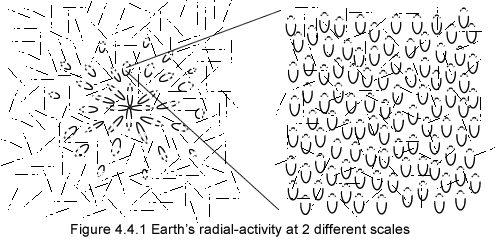A copy of figure 4.3, which represents the whole of Earths radial-activity, is reproduced on the left side of figure 4.4.1.
Now let us consider in the sky above us, a volume of Earths radial-activity that is only a very small portion of all of Earths radial activity.
Represented on the right side of the figure is such a small yet enlarged illustration of it. And rather than being uniform (as shown) the motion-occurrences on the right side that are closer to the ground have more thrust. And instead of being parallel (as shown) all of these MOs are actually converging toward the center of Earth.
In figure 4.4.2 below the right side of figure 4.4.1 is now shown on the left, while the radial-activity of a meteorite is drawn on the right. For simplicity the background pure-activity is omitted, as it has no effect; and while far away from any gravitational field including that of Earth, the meteorite is supposed to have no overall motion and it is represented as such (with no overall motion) that is with a smaller and symmetrical yet dynamic radial activity.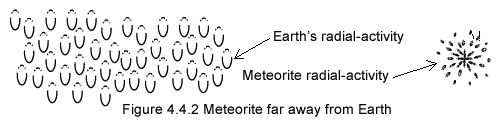Finally the meteorite finds itself within the scope of Earths radial activity (gravitation) through the motion of the entire solar system, which is moving toward the meteorite.
As soon as Earth's radial activity overlaps the meteorite's radial-activities the 2 interact and modify each other as described below: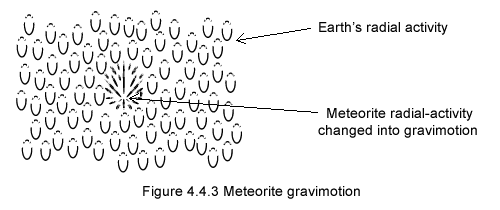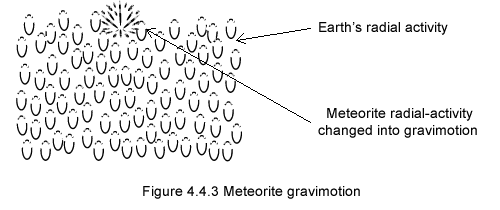The meteorites radial-activity is thrown out of balance and to a lesser degree Earths radial activity is too. When sharing Earths radial-activity, the meteorites own radial-activity loses its symmetry, it becomes gravimotion and that amounts to the very motion of the meteorite due to the gravitation surrounding Earth; the meteorite falling toward Earth can then be represented with a motion-occurrence as shown in these models. Make sure to hover your mouse pointer over the illustrations.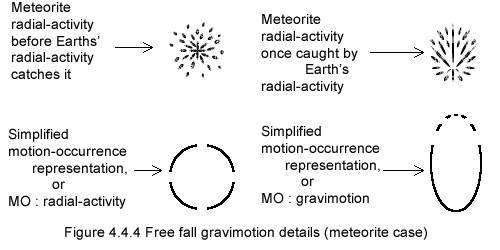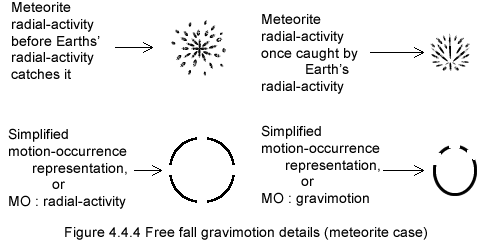As one can see the model representing the motion (free fall on the right) of the meteorite, or any other object in free fall, is similar to that of a
motion-occurrence §1.3
Reminder:
Motion model or the motion-occurrence (§1.3)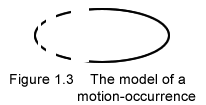, but for the fact that on the right of Figure 4.4.4 that motion (gravimotion) is accelerating and inclined toward the ground, while the MO of figure 1.3 is moving horizontally.

As a general rule any material object in motion is in motion through a modification of its inner motion constitution that is a modification of its radial-activity.
No matter the cause of motion,
any motion animating matter is radial-activity turned into gravimotion.
Gravimotion's pragmatism versus physics faultless mathematics
1. Both Newton and Einstein interpretations of gravitation are based on traditional assumptions
1. The mass of any material body generates both Newton's gravitation force and Einstein's space-time curvature.
2. Newton's force and Einstein's curvature, even though directed backward, have no effect on the mass that engenders them.
3. Both force and curvature have a direct effect on all body masses of the universe but their own.
2. Gravimotion is based on the reality of motion
1. Gravitation is motion that comes right out of space, and not a force coming out of mass as in physics interpretation item 1 above.
2. Gravitation turned into gravimotion puts in motion the matter it is directed to, the opposite of physics interpretation item 2 above.
3. Earths surrounding radial-activity (physics' gravitational field) interacts with Newton's apple own radial-activity and not with the apple's mass as in physics. Here too, the assumption that gravitation acts on gravitation and not on mass departs drastically from physics interpretation item 3 above.

And as usual all the MOs of the model are animated with their subjacent motions and in addition are also jiggling around; motions that are for most of them not represented here.

In the end the motion of the meteorite is controlled by Earths radial activity and not by Earths mass. That is a drastic departure from conventional thinking; it liberates for all purposes our concept of gravitation from mass.
Gravimotions interpretation of gravitation doesn't require our mental concept of mass!

A falling object's motion and its radial-activity make one hence the word gravimotion; a phenomenon akin to Einstein's time dilation (§1.10), as both time dilation and gravimotion are the effect of 2 interacting motions.
Einstein went a step farther showing mathematically that gravitational field interacts with light; in gravimotion both radial-activity and light (§1.2) are pure motions, and in Einstein's footsteps, when overlapping do interact.